# Determine the type of operating system using VBA in Microsoft Excel

To identify the type of OS, we can use macro code. In this article we will learn how to determine the type of operating system being used using VBA code.

Question: I would like to know the OS of my system through VBA code whether it is 32 bit or 64 bit.

To determine the type of operating system we need to follow the below steps:

• We need to launch VB editor
• Click on Developer tab
• From Code group select Visual Basic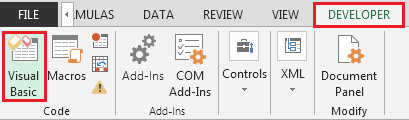• Click on Insert then Module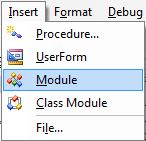• This will create new module
• Enter the following code in the Module

```Function OSis32BIT() As Boolean
OSis32BIT = False
If InStr(Application.OperatingSystem, "32-bit") Then
OSis32BIT = True
End If
End Function
```

```Function OSis64BIT() As Boolean
OSis64BIT = False
If InStr(Application.OperatingSystem, "64-bit") Then
OSis64BIT = True
End If
End Function
```

```Sub TestOSis32BIT()
If OSis32BIT Then
MsgBox "You use a 32bit operating system", , _
Application.OperatingSystem
Else
MsgBox "You don't use a 32bit operating system", , _
Application.OperatingSystem
End If
End Sub

```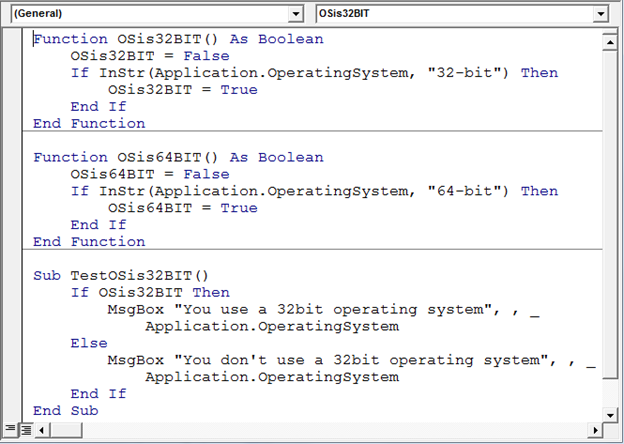As you run the macro you will get a message box, refer to the below snapshot: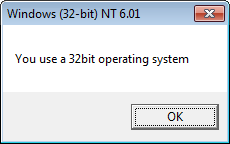If you are using 64 bit operating system then the message box will update "You don't use a 32bit operating system"

To check whether the system is 32 bit; we can use UDF in an empty cell as:

• =OSis32BIT(); this will return TRUE if operating system is 32 bit otherwise return FALSE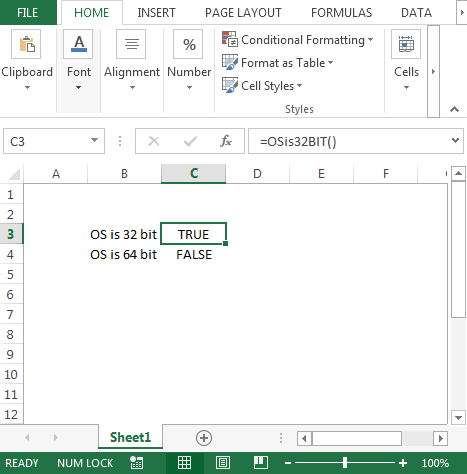To check whether the system is 64 bit; we can use UDF in an empty cell as:

• =OSis64BIT(); this will return TRUE if operating system is 64 bit otherwise return FALSE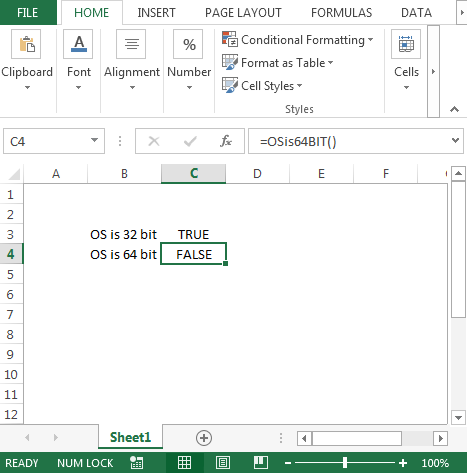Conclusion: In this way using VBA code, we can identify the operating system we are using.We would love to hear from you, do let us know how we can improve, complement or innovate our work and make it better for you. Write us at info@exceltip.com

1.